﻿ 不同市场权力结构下零售商双渠道供应链中定价决策研究 Pricing Decisions in a Dual Channel Supply Chain with Different Market Power Structures

Operations Research and Fuzziology
Vol.08 No.02(2018), Article ID:25165,10 pages
10.12677/ORF.2018.82008

Pricing Decisions in a Dual Channel Supply Chain with Different Market Power Structures

Jiajing Dong, Jing Zhao*, Yunlian Guo

School of Science, Tianjin Polytechnic University, Tianjin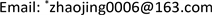Received: May 8th, 2018; accepted: May 19th, 2018; published: May 29th, 2018ABSTRACT

This paper investigates the pricing decision problem of a dual-channel supply chain consisting of one manufacturer and one retailer which opens an online channel. From the perspective of consumer utility, and considering the difference of market power among members of supply chain and the difference of relative channel power, three pricing game models are established, and the corresponding analytic equilibriums are obtained. Furthermore, we analyze the influence of the market power structure, channel substitutability and the relative channel power on the pricing strategies, the maximal demands and the maximal profits.

Keywords:Dual-Channel Supply Chain, Channel Power, Pricing Decision, Consumer UtilityCopyright © 2018 by authors and Hans Publishers Inc.1. 引言

2. 问题描述及假设

$U=\sum _{i=1}^{2}\left({\alpha }_{i}{D}_{i}-\frac{1}{2}{D}_{i}^{2}\right)-\theta {D}_{1}{D}_{2}-\sum _{i=1}^{2}{p}_{i}{D}_{i},$ (1)

${D}_{1}=\frac{{\alpha }_{1}-\theta {\alpha }_{2}-{p}_{1}+\theta {p}_{2}}{1-{\theta }^{2}},$ (2)

${D}_{\text{2}}=\frac{{\alpha }_{\text{2}}-\theta {\alpha }_{\text{1}}-{p}_{\text{2}}+\theta {p}_{\text{1}}}{1-{\theta }^{2}}.$ (3)

${\pi }_{m}\left(w\right)=\left({D}_{1}+{D}_{2}\right)w,$ (4)

${\pi }_{r}\left({p}_{1},{p}_{2}\right)={D}_{1}\left({p}_{1}-w-{c}_{1}\right)+{D}_{2}\left({p}_{2}-w-{c}_{2}\right).$ (5)

3. 模型建立及求解

3.1. 制造商主导的Stackelberg (MS)博弈模型

$\left\{\begin{array}{l}\underset{w}{\mathrm{max}}{\pi }_{m}\left(w,{p}_{1}\left(w\right),{p}_{2}\left(w\right)\right)\\ 对以下问题求解得到\text{\hspace{0.17em}}{p}_{1}\left(w\right),{p}_{2}\left(w\right)\\ \underset{\left({p}_{1},{p}_{2}\right)}{\mathrm{max}}{\pi }_{r}\left({p}_{1},{p}_{2}\right)\end{array}.$ (6)

${w}^{M*}=\frac{\left({\alpha }_{1}-{c}_{1}\right)+\left({\alpha }_{2}-{c}_{2}\right)}{4},$ (7)

${p}_{1}^{M*}=\frac{\left(5{\alpha }_{1}+3{c}_{1}\right)+\left({\alpha }_{2}-{c}_{2}\right)}{8},$ (8)

${p}_{\text{2}}^{M*}=\frac{\left({\alpha }_{1}-{c}_{1}\right)+\left(5{\alpha }_{2}+3{c}_{2}\right)}{8}.$ (9)

3.2. 零售商主导的Stackelberg (RS)博弈模型

$\left\{\begin{array}{l}\underset{\left({p}_{1},{p}_{2}\right)}{\mathrm{max}}{\pi }_{r}\left({p}_{1},{p}_{2},w\left({p}_{1},{p}_{2}\right)\right)\\ 对以下问题求解得到\text{\hspace{0.17em}}w\left({p}_{1},{p}_{2}\right)\\ \underset{w}{\mathrm{max}}{\pi }_{m}\left(w\right)\end{array}.$ (10)

${w}^{R*}=\frac{\left({\alpha }_{1}-{c}_{1}\right)+\left({\alpha }_{2}-{c}_{2}\right)}{8},$ (11)

${p}_{1}^{R*}=\frac{\left(5{\alpha }_{1}+3{c}_{1}\right)+\left({\alpha }_{2}-{c}_{2}\right)}{8},$ (12)

${p}_{2}^{R*}=\frac{\left({\alpha }_{1}-{c}_{1}\right)+\left(5{\alpha }_{2}+3{c}_{2}\right)}{8}.$ (13)

3.3. 纳什博弈(NG)模型

$\left\{\begin{array}{l}\underset{\left({p}_{1},{p}_{2}\right)}{\mathrm{max}}{\pi }_{r}\left({p}_{1},{p}_{2}\right)\\ \underset{w}{\mathrm{max}}{\pi }_{m}\left(w\right)\end{array}.$ (14)

${w}^{N*}=\frac{\left({\alpha }_{1}-{c}_{1}\right)+\left({\alpha }_{2}-{c}_{2}\right)}{\text{6}},$ (15)

${p}_{1}^{N*}=\frac{\left(\text{7}{\alpha }_{1}+\text{5}{c}_{1}\right)+\left({\alpha }_{2}-{c}_{2}\right)}{\text{12}},$ (16)

${p}_{2}^{N*}=\frac{\left({\alpha }_{1}-{c}_{1}\right)+\left(\text{7}{\alpha }_{2}+\text{5}{c}_{2}\right)}{\text{12}}.$ (17)

4. 模型分析与管理启示

4.1. 不同博弈模型下均衡解比较

${D}^{\text{*}}={D}_{1}^{*}+{D}_{2}^{*}$${\pi }^{\text{*}}={\pi }_{m}^{*}+{\pi }_{r}^{*}$$A=\frac{{\left({\alpha }_{2}-{c}_{2}\right)}^{2}}{1-{\theta }^{2}}$$B={\Omega }^{2}+1$Table 1. Maximum demand and profit under different game models

4.2. 渠道替代率对最大需求、最大利润和消费者效用的影响

1) $\frac{\partial {D}_{i}^{N*}}{\partial \theta }<\frac{\partial {D}_{i}^{M*}}{\partial \theta }=\frac{\partial {D}_{i}^{R*}}{\partial \theta }$$i=1,2,\cdots$$\frac{\partial {D}^{T*}}{\partial \theta }<0$ ，其中 $T=M或R或N$

2) $\frac{\partial {D}_{\text{1}}^{S\text{*}}}{\partial \theta }\left\{\begin{array}{c}>0,如果\frac{3{\theta }^{2}+2\theta +3}{{\theta }^{2}+6\theta +1}<\Omega <\frac{3+\theta }{1+3\theta }\\ <0,如果\frac{1+3\theta }{3+\theta }<\Omega <\frac{3{\theta }^{2}+2\theta +3}{{\theta }^{2}+6\theta +1}\end{array}$ ，其中 $S=M或R$

$\frac{\partial {D}_{\text{2}}^{S\text{*}}}{\partial \theta }\left\{\begin{array}{c}>0,如果\frac{{\theta }^{2}+6\theta +1}{3{\theta }^{2}+2\theta +3}<\Omega <\frac{3+\theta }{1+3\theta }\\ <0,如果\frac{1+3\theta }{3+\theta }<\Omega <\frac{{\theta }^{2}+6\theta +1}{3{\theta }^{2}+2\theta +3}\end{array}$ ，其中 $S=M或R$

3) $\frac{\partial {D}_{\text{1}}^{N\text{*}}}{\partial \theta }\left\{\begin{array}{c}>0,如果\frac{5{\theta }^{2}+2\theta +5}{{\theta }^{2}+10\theta +1}<\Omega <\frac{5+\theta }{1+5\theta }\\ <0,如果\frac{1+5\theta }{5+\theta }<\Omega <\frac{5{\theta }^{2}+2\theta +5}{{\theta }^{2}+10\theta +1}\end{array}$

1) 随着渠道替代率的增大，两个渠道的最大需求均增大。具体来说，在区域 ${A}_{1}$ 中， $\frac{\partial {D}_{\text{1}}^{S\text{*}}}{\partial \theta }>0$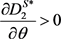；在区域 ${A}_{2}$ 中， $\frac{\partial {D}_{\text{1}}^{N\text{*}}}{\partial \theta }>0$$\frac{\partial {D}_{\text{2}}^{N\text{*}}}{\partial \theta }>0$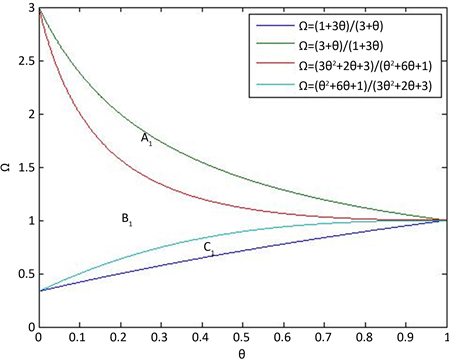Figure 1. Maximum demand for channels change with θ (Stackelberg game)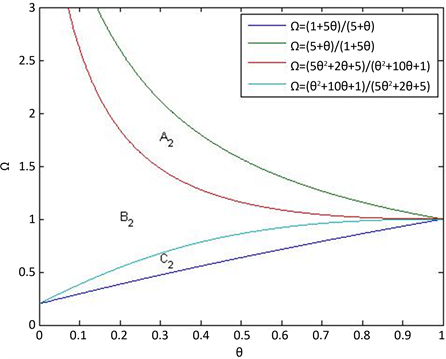Figure 2. Maximum demand for channels change with θ (Nash game)

2) 随着渠道替代率的增大，直销渠道的最大需求减小，零售渠道的最大需求增大。在区域 ${B}_{1}$ 中， $\frac{\partial {D}_{\text{1}}^{S\text{*}}}{\partial \theta }<0$$\frac{\partial {D}_{\text{2}}^{S\text{*}}}{\partial \theta }>0$ ；在区域 ${B}_{2}$ 中， $\frac{\partial {D}_{\text{1}}^{N\text{*}}}{\partial \theta }<0$

3) 随着渠道替代率的增大，渠道的最大需求减小。在区域 ${C}_{1}$ 中， $\frac{\partial {D}_{\text{1}}^{S\text{*}}}{\partial \theta }<0$$\frac{\partial {D}_{\text{2}}^{S\text{*}}}{\partial \theta }<0$ ；在区域 ${C}_{2}$ 中， $\frac{\partial {D}_{\text{1}}^{N\text{*}}}{\partial \theta }<0$$\frac{\partial {D}_{\text{2}}^{N\text{*}}}{\partial \theta }<0$

5. 结论与展望

Pricing Decisions in a Dual Channel Supply Chain with Different Market Power Structures[J]. 运筹与模糊学, 2018, 08(02): 62-71. https://doi.org/10.12677/ORF.2018.82008

1. 1. Hsiao, L. and Chen, Y. (2014) Strategic Motive for Introducing Internet Channels in a Supply Chain. Production & Operations Management, 23, 36-47. https://doi.org/10.1111/poms.12051

2. 2. Wang, W., Li, G. and Cheng, T.C.E. (2016) Channel Selection in a Supply Chain with a Multi-Channel Retailer: The Role of Channel Operating Costs. International Journal of Production Economics, 173, 54-65. https://doi.org/10.1016/j.ijpe.2015.12.004

3. 3. King, R.C., Sen, R. and Xia, M. (2004) Impact of Web-Based E-Commerce on Channel Strategy in Retailing. International Journal of Electronic Commerce, 8, 103-130.

4. 4. Liu, X.F. and Qian, X.D. (2017) Product Brand Differentiation and Dual-Channel Store Performances of a Multi-Channel Retailer. Education Teaching Forum, 44, 672-692.

5. 5. Bernsteina, F. and Zheng, X. (2008) “Bricks-and-Mortar” vs. “Clicks-and-Mortar”: An Equilibrium Analysis. European Journal of Operational Research, 187, 671-690. https://doi.org/10.1016/j.ejor.2006.04.047

6. 6. Shi, R., Zhang, J. and Ru, J. (2015) Impacts of Power Structure on Supply Chains with Uncertain Demand. Production &Operations Management, 20 Contracts for Fresh Product Outsourcing Logistics Channels with Power Structures. International Journal of Production Economics, 160, 94-105. https://doi.org/10.1016/j.ijpe.2014.10.007

7. 7. Wu, Q., Mu, Y. and Feng, Y. (2015) Coordinating Channel Selection Decisions under Different Power Structures. Omega, 53, 11-20.

8. 8. Chen, X. and Wang, X. (2015) Free or Bundled: Channel Selection Decisions under Different Power Structures. Omega, 53, 11-20. https://doi.org/10.1016/j.omega.2014.11.008

9. 9. Wei, J., Zhao, J. and Li, Y. (2013) Pricing Decisions for Complementary Products with Firms’ Different Market Powers. European Journal of Operational Research, 224, 507-519. https://doi.org/10.1016/j.ejor.2012.09.011

10. 10. Luo, Z., Chen, X., Chen, J., et al. (2016) Optimal Pricing Policies for Differentiated Brands under Different Supply Chain Power Structures. European Journal of Operational Research, 259, 437-451. https://doi.org/10.1016/j.ejor.2016.10.046

11. 11. Cai, G. (2010) Channel Selection and Coordination in Dual-Channel Supply Chains. Journal of Retailing, 86, 22-36. https://doi.org/10.1016/j.jretai.2009.11.002

12. 12. Cai, G., Dai, Y. and Zhou, S.X. (2012) Exclusive Channels and Revenue Sharing in a Complementary Goods Market. Marketing Science, 31, 172-187. https://doi.org/10.1287/mksc.1110.0688

13. 13. Zhang, R., Liu, B., Wang, W., et al. (2012) Pricing Decisions in a Dual Channels System with Different Power Structures. Economic Modelling, 29, 523-533. https://doi.org/10.1016/j.econmod.2011.08.024

$\frac{\partial {\pi }_{r}}{\partial {p}_{1}}=\frac{1}{1-{\theta }^{2}}\left(2\theta {p}_{2}-2{p}_{1}+\left(1-\theta \right)w+\left({\alpha }_{1}+{c}_{1}\right)-\theta \left({\alpha }_{2}+{c}_{2}\right)\right),$ (a1)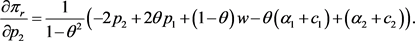(a2)

$\left(\begin{array}{cc}\frac{{\partial }^{\text{2}}{\pi }_{r}}{\partial {p}_{2}^{2}}& \frac{{\partial }^{2}{\pi }_{r}}{\partial {p}_{2}\partial {p}_{1}}\\ \frac{{\partial }^{2}{\pi }_{r}}{\partial {p}_{1}\partial {p}_{2}}& \frac{{\partial }^{\text{2}}{\pi }_{r}}{\partial {p}_{1}^{2}}\end{array}\right)=\frac{4}{{\left(1-{\theta }^{2}\right)}^{2}}\left(\begin{array}{cc}-1& \theta \\ \theta & -1\end{array}\right),$ (a3)

$\text{0}\le \theta <1$ ，Hessian矩阵负定，因此式(5)是 ${p}_{1}$${p}_{2}$ 的凹函数，令式(a1)、(a2)为零，解得

${p}_{\text{1}}\left(w\right)=\frac{1}{2}\left(w+{\alpha }_{\text{1}}+{c}_{\text{1}}\right),$ (a4)

${p}_{2}\left(w\right)=\frac{1}{2}\left(w+{\alpha }_{2}+{c}_{2}\right).$ (a5)

${\pi }_{m}\left(w\right)$$w$ 求一阶和二阶偏导数得：

$\frac{\text{d}{\pi }_{m}}{\text{d}w}=\frac{1}{1-\theta }\left(-w+\frac{1}{4}\left({\alpha }_{1}-{c}_{1}\right)+\frac{1}{4}\left({\alpha }_{2}-{c}_{2}\right)\right),$ (a6)

$\frac{{\text{d}}^{\text{2}}{\pi }_{m}}{\text{d}{w}^{2}}=-\frac{1}{1+\theta }<0.$ (a7)

${p}_{i}=w+{m}_{i},\left(i=1,2\right),$ (a8)

${\pi }_{m}\left(w\right)$$w$ 求一阶和二阶偏导数得：：

$\frac{\text{d}{\pi }_{m}}{\text{d}w}=\frac{{\alpha }_{\text{1}}+{\alpha }_{\text{2}}-{m}_{1}-{m}_{2}-4w}{1-\theta },$ (a9)

$\frac{{\text{d}}^{\text{2}}{\pi }_{m}}{\text{d}{w}^{2}}=-\frac{\text{4}}{1+\theta }<0,$ (a10)

$w=\frac{{\alpha }_{1}+{\alpha }_{2}-{p}_{1}-{p}_{2}}{2},$ (a11)

${\pi }_{r}\left({p}_{1},{p}_{2}\right)$ 分别对 ${p}_{1}$${p}_{2}$ 求一阶偏导数得：

$\frac{\partial {\pi }_{r}}{\partial {p}_{1}}=\frac{1}{1-{\theta }^{2}}\left[\left(\theta -3\right){p}_{1}+\left(3\theta -1\right){p}_{2}-\left(2\theta -1\right){\alpha }_{2}-\left(\theta -2\right){\alpha }_{1}-{c}_{2}\theta +{c}_{1}\right],$ (a12)

$\frac{\partial {\pi }_{r}}{\partial {p}_{2}}=\frac{1}{1-{\theta }^{2}}\left[\left(\theta -3\right){p}_{\text{2}}+\left(3\theta -1\right){p}_{\text{1}}-\left(2\theta -1\right){\alpha }_{\text{1}}-\left(\theta -2\right){\alpha }_{\text{2}}-{c}_{\text{1}}\theta +{c}_{\text{2}}\right],$ (a13)

$\left(\begin{array}{cc}\frac{{\partial }^{\text{2}}{\pi }_{r}}{\partial {p}_{2}^{2}}& \frac{{\partial }^{2}{\pi }_{r}}{\partial {p}_{2}\partial {p}_{1}}\\ \frac{{\partial }^{2}{\pi }_{r}}{\partial {p}_{1}\partial {p}_{2}}& \frac{{\partial }^{\text{2}}{\pi }_{r}}{\partial {p}_{1}^{2}}\end{array}\right)=\frac{\text{1}}{{\left(1-{\theta }^{2}\right)}^{2}}\left(\begin{array}{cc}\theta -3& 3\theta -1\\ 3\theta -1& \theta -3\end{array}\right),$ (a14)

$\text{0}\le \theta <1$ ，Hessian矩阵负定，式(5)是 ${p}_{1}$${p}_{2}$ 的凹函数，令式(a12)、(a13)为零，得式(12)和(13)。将式(12)和(13)代入(a11)，得(11)。将(11)~(13)式代入(2)~(5)式得渠道最大需求和最大利润。